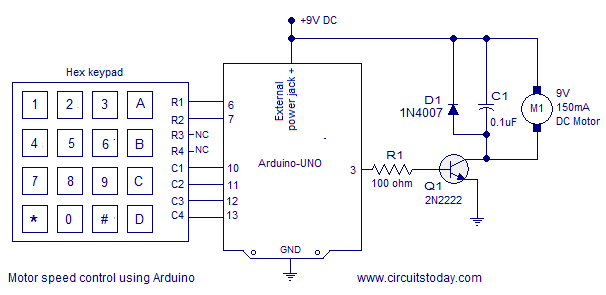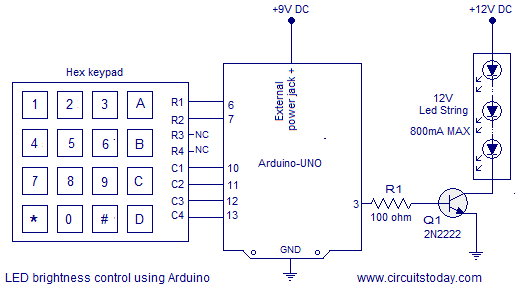## Audio

[Audio][slideshow]

## PWM motor speed control using Arduino.

PWM or pulse width modulation is a very common method used for controlling the power across devices like motor, light etc. In PWM method the power across the load is controlled by varying the duty cycle of the drive signal. More the duty cycle more power is delivered across the load and less the duty cycle, less power is delivered across the load. A hex keypad is used for controlling the speed. The speed can be varied in seven steps using the hex keypad. Arduino UNO is the type os arduino development board used in this circuit. The circuit diagram of the PWM motor speed control using arduino is shown in the figure below.

### Circuit diagram.Row pins R1 and R2 of the hex keypad are interfaced to digital pins 6 and 7 of the arduino. Column pins C1, C2, C3 and C4 are interfaced to the digital pind 10, 11, 12 and 13 of the arduino. The key pressed on the hex keypad is identified using the column scanning method and it is explained in detail in this article. Interfacing hex keypad to arduino. The digital pins of the arduino can source or sink only up to 4omA of current. So the digital pin 3 cannot drive the motor directly. To solve this problem an NPN transistor (2N2222) is used to drive the motor according the the PWM signal available at digital pin 3. 100 ohm resistor R1 is used to limit the base current of the transistor. The motor is connected as a collector load to the transistor. The 0.1uF capacitor C1 connected across the motor is used to by-pass the voltage spikes and noises produced during the switching of the motor.

The arduino board is powered through the external power jack provided on the board. The arduino board can be also powered by the PC through USB but there must be an additional external source for powering the motor. The complete program for PWM motor speed control using arduino is given below. Explanation of the program is given under the “About the program” heading.

### Program.

`int pwm=3; // declares digital pin 3 as PWM output  int r1=6;  int r2=7;  int c1=10;  int c2=11;  int c3=12;  int c4=13;  int colm1;  int colm2;  int colm3;  int colm4;    void setup()  {    pinMode(r1,OUTPUT);    pinMode(r2,OUTPUT);    pinMode(c1,INPUT);    pinMode(c2,INPUT);    pinMode(c3,INPUT);    pinMode(c4,INPUT);    pinMode(pwm,OUTPUT);    digitalWrite(c1,HIGH);    digitalWrite(c2,HIGH);    digitalWrite(c3,HIGH);    digitalWrite(c4,HIGH);    digitalWrite(pwm,LOW);  }  void loop()  {    digitalWrite(r1,LOW);    digitalWrite(r2,HIGH);    colm1=digitalRead(c1);    colm2=digitalRead(c2);    colm3=digitalRead(c3);    colm4=digitalRead(c4);    if(colm1==LOW)         //checks whether key "1" is pressed.    { analogWrite(pwm,42); // writes "42" (duty cycle 16%).     delay(200);}    else    {     if(colm2==LOW)        //checks whether key "2" is pressed.     { analogWrite(pwm,84); // writes "84" (duty cycle 32%).      delay(200);}     else     {     if(colm3==LOW)        //checks whether key "3" is pressed     {analogWrite(pwm,126); // writes "126" (duty cycle 48%).     delay(200);}     else     {     if(colm4==LOW)        // checks whether key"A" is pressed.     {digitalWrite(pwm,LOW); // makes pin 3 LOW (duty cycle 0%).Motor OFF.      delay(200);}     }}}      digitalWrite(r1,HIGH);    digitalWrite(r2,LOW);    colm1=digitalRead(c1);    colm2=digitalRead(c2);    colm3=digitalRead(c3);    colm4=digitalRead(c4);    if(colm1==LOW)         // checks whether key "4" is pressed.    {analogWrite(pwm,168); //writes "168" (duty cycle 64%).    delay(200);}    else    {     if(colm2==LOW)        // checks whether key "5" is pressed.     {analogWrite(pwm,202); // writes "202" (duty cycle 80%).    delay(200);}     else     {     if(colm3==LOW)        // checks whether key "6" is pressed.     {analogWrite(pwm,244); // writes "244" (duty cycle 96%).      delay(200);}     else     {     if(colm4==LOW)          // checks whether key "B" is pressed.     {digitalWrite(pwm,HIGH);//makes pin 3 HIGH (duty cycle 100%). FULL POWER     delay(200); }       }}}}`

The duty cycle of the PWM control signal is varied by varying the value written to the output pin 3 using the analogWrite() function. The range of the value that can be written is between 0 and 255. The anlogWrite() function can be employed on pins 3, 5, 6, 9, 10 and 11 in the Arduino UNO board. In most of the arduino boards the frequency of the PWM signal will be around 490Hz. The duty cycle of the PWM signal is proportional to the value written using the analogWrite() function. Few examples using the analogWrite() function are shown below.

• analogWrite(pwm,255) will generate a pwm wave of 100% duty cycle (full power) at the pin denoted by the variable “pwm”.
• analogWrite(pwm,128) will generate a pwm wave of 50% duty cycle (half power) at the pin denote by the variable “pwm”.
• analogWrite(pwm,0) will generate a pwm wave of 0% duty cycle (no power) at the pin denoted by the variable “pwm”.

In the program the digital pin 3 is configured as the PWM output pin. Keys 1 to 6 on the hex keypad are used for increasing the power in steps of “42” in terms of the value written using the analogWrite() function or 16% in terms of duty cycle. Key “A” on the hex keypad is used for switching the motor OFF and it is done using the command “digitalWrite(pwm,LOW);. Key “B” on the hex keypad is used for putting the motor at maximum speed an it is done using the command “digitalWrite(pwm,HIGH);.

#### Notes.

Instead the motor you can also use the same circuit for varying the brightness of an LED string. Any way the load current must be in the safe limits of transistor 2N2222 and it is 800mA. Also the external power supply must be powerful enough to drive the LED string. The circuit diagram of PWM brightness control of LED using arduino is shown in the figure below.[Lights][grids]